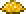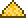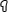•类型 \l!--\n --\g\{\{#vardefine:_typepage\!\{\{#or:\{\{l10n\!item_infobox\!typepage:@@@@\}\}\!\{\{tr\!\{\{ucfirst:@@@@\}\}\!link\ey\}\}\}\}\}\}\l!--\_\n --\g\{\{#vardefine:_typetext\!\{\{#or:\{\{l10n\!item_infobox\!type:@@@@\}\}\!\{\{tr\!\{\{ucfirst:@@@@\}\}\}\}\}\}\}\}\l!--\_\n --\g\{\{nowrap\!class\etag\!\{\{ifexists\!\{\{#var:_typepage\}\}\!\(\(\{\{#var:_typepage\}\}\!\{\{#var:_typetext\}\}\)\)\!\{\{#var:_typetext\}\}\}\}\}\}\l!--\n --\g1 需要 25 份掉落自经典专家大师

## 制作

### 用于

(10) ()徒手
(5) ('"`UNIQ--esc-0000001D-QINU`"' '"`UNIQ--esc-0000001E-QINU`"')
()

('"`UNIQ--esc-0000001D-QINU`"' '"`UNIQ--esc-0000001E-QINU`"')
(\(\(Category:Hardmode-only\_NPCs\{\{#switch:\{\{lc:\$type\$\}\}\!npc\!critter\e\{\{!\}\}*\}\}\)\) \(\(Category:Expert\_mode\_exclusive\_NPCs\{\{#switch:\{\{lc:\$type\$\}\}\!npc\!critter\e\{\{!\}\}*\}\}\)\) \(\(Category:\{\{tr\!\{\{#switch:\{\{lc:\$env\$\}\}\!meteorite\_(biome)\eMeteorite\!#default\e\$env\$\}\}\_\}\}\_NPC\!\{\{PAGENAME\}\}\)\) \(\(Category:\{\{#switch:\{\{lc:\$type\$\}\}\!background\_object\e\{\{tr\!Background\_object\_items\}\}\!#default\e\{\{tr\!\$type\$\}\}\_NPC\}\}\!\{\{PAGENAME\}\}\)\) \(\(Category:\{\{tr\!\$ai\$\}\}\_NPC\!\{\{PAGENAME\}\}\)\) \(\(Category:\{\{tr\!\$listcat\$\}\}\_NPC\!\{\{PAGENAME\}\}\)\))
(\(\(Category:Expert\_mode\_exclusive\_NPCs\{\{#switch:\{\{lc:\$type\$\}\}\!npc\!critter\e\{\{!\}\}*\}\}\)\))
(200) ()
(45) ('"`UNIQ--esc-0000001D-QINU`"' '"`UNIQ--esc-0000001E-QINU`"')
()
('"`UNIQ--esc-0000001D-QINU`"' '"`UNIQ--esc-0000001E-QINU`"')
(3) ()

('"`UNIQ--esc-0000001D-QINU`"' '"`UNIQ--esc-0000001E-QINU`"')
total: 13 row(s)

## 备注

• 最好的将多余的妖精尘变现的方式是制作强效治疗药水。一组 99 个妖精尘，33 个水晶加上 100 块沙/50 块玻璃 就可以比材料的卖价（卖价主要来源于妖精尘）多赚363 (58%)。若使用炼药桌，则可多产出 50%（额外赚大约5）。
• 翅膀也很有赚头，但各种"翅膀核心材料"（用于制作翅膀）为飞翔之魂提供了竞争。